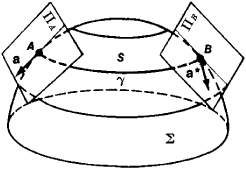# Parallel Displacement

## parallel displacement

[′par·ə‚lel di′splās·mənt]
(mathematics)
A vector A at a point P of an affine space is said to be obtained from a vector B at a point Q of the space by a parallel displacement with respect to a curve connecting A and B if a vector V (X) can be associated with each point X on the curve in such a manner that A = V (P), B = V (Q), and the values of V at neighboring points of the curve are parallel as specified by the affine connection.
McGraw-Hill Dictionary of Scientific & Technical Terms, 6E, Copyright © 2003 by The McGraw-Hill Companies, Inc.
The following article is from The Great Soviet Encyclopedia (1979). It might be outdated or ideologically biased.

## Parallel Displacement

a generalization of the concept of translation to spaces with a more complex structure than Euclidean spaces, for example, affinely connected spaces and, in particular, Riemannian spaces. Parallel displacement permits the comparison of geometric figures associated with different points of a space.

A parallel displacement on a surface Σ in three-dimensional Euclidean space, where Σ is a two-dimensional Riemannian space, is defined as follows. Let γ be a curve on Σ, let A and B be the endpoints of y, let S be the developable surface that is the envelope of the family of tangent planes at points of y, and let a be a vector at the point A in the tangent plane πA (see Figure 1). When S is developed on a plane, the translation of a in S with a subsequent adjoining of S to γ is called the parallel dislacement of a. The vector a* in Figure 1 is the result of a parallel displacement of a along γ in Σ.Figure 1

Parallel displacement can be regarded as a linear transformation of a tangent plane πA at a point A into a tangent plane ΠB at a point B. Such a transformation can be described by formulas expressed in terms of Christoffel symbols. These formulas can be generalized to n-dimensional Riemannian spaces and to affinely connected spaces; the Christoffel symbols can accordingly be calculated through the use of a metric tensor or be given as the initial parameters of the theory.

In general, the result of the parallel displacement of a vector depends not only on the initial vector and the initial and final displacement points but also on the choice of the displacement path. If the result of the parallel displacement of a vector is independent of the choice of the path, the space (at least within a sufficiently small neighborhood) is affine or Euclidean, and parallel displacement coincides with translation.

D. D. SOKOLOV